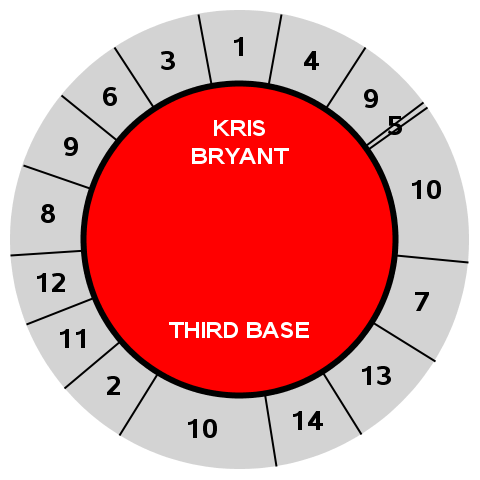## Fun With SAS ODS Graphics: All-Star Baseball-like Donut Charts

SAS OUTPUTSeeing Chevell Parker pull-donut-charts-out-of-a-SAS-Excel-hat at SASGF 2017 made me try to recall, "When did I see a donut chart for the first time?" Well, SAS/GRAPH users have had donut charts in their bag-of-dataviz-tricks for about a quarter of a century. But that wasn't it. Even earlier users of donut charts, including me, were kids who grew up playing the sadly-discontinued Cadaco All-Star Baseball board game, where pie-charts-with-a-hole-in-the-middle were used to present MLB batting stats on game discs since 1941. So, after reading Prashant Hebbar and Sanjay Matange's SASGF paper on Easy Polar Graphs with SG Procedures, I thought to myself, "Could I use SAS ODS Graphics to create All-Star Baseball disc knock-offs?"

SAS CODE

``````* Fun with SAS ODS Graphics: Knock-off of All-Star Baseball "donut chart" discs;

data mlbstats;                                             * Create disc categories from MLB stats;
infile cards dlm='|' dsd firstobs=2;                       * Read MLB stats;
input Player : \$30. Pos : \$20. Year Team : \$3. LG : \$2. G AB R H TB _2B _3B HR RBI BB IBB SO SB CS AVG OBP SLG OPS GO_AO;
PlayerNum+1;                                               * Assign player sequence #;
player=upcase(player);                                     * Player name;
pos=upcase(pos);                                           * Player position;
wedgeID=01; stat=HR; output;                               * Home run;
wedgeID=02; stat=(AB-H-SO)*GO_AO/(GO_AO+1)/3; output;      * Ground out I;
wedgeID=03; stat=(AB-H-SO)*(1-GO_AO/(GO_AO+1))/4; output;  * Fly out I;
wedgeID=04; stat=(AB-H-SO)*(1-GO_AO/(GO_AO+1))/4; output;  * Fly out II;
wedgeID=05; stat=_3B; output;                              * Triple;
wedgeID=06; stat=(AB-H-SO)*GO_AO/(GO_AO+1)/3; output;      * Ground out II;
wedgeID=07; stat=(H-HR-_3B-_2B)/2; output;                 * Single I;
wedgeID=08; stat=(AB-H-SO)*(1-GO_AO/(GO_AO+1))/4; output;  * Fly out III;
wedgeID=09; stat=BB/2; output;                             * Walk I;
wedgeID=09; stat=BB/2; output;                             * Walk II;
wedgeID=10; stat=SO/2; output;                             * Strikeout I;
wedgeID=10; stat=SO/2; output;                             * Strikeout II;
wedgeID=11; stat=_2B; output;                              * Double;
wedgeID=12; stat=(AB-H-SO)*GO_AO/(GO_AO+1)/3; output;      * Ground out III;
wedgeID=13; stat=(H-HR-_3B-_2B)/2; output;                 * Single II;
wedgeID=14; stat=(AB-H-SO)*(1-GO_AO/(GO_AO+1))/4; output;  * Fly out IV;
cards;                                                     * 2016-17 MLB Cubs batting stats (source: mlb.com);
Player|Pos|Year|Team|LG|G|AB|R|H|TB|2B|3B|HR|RBI|BB|IBB|SO|SB|CS|AVG|OBP|SLG|OPS|GO_AO|
Kris*Bryant|Third Base|2016|CHC|NL|155|603|121|176|334|35|3|39|102|75|5|154|8|5|0.292|0.385|0.554|0.939|0.59|
Anthony*Rizzo|First Base|2016|CHC|NL|155|583|94|170|317|43|4|32|109|74|8|108|3|5|0.292|0.385|0.544|0.928|0.91|
Dexter*Fowler|Center Field|2016|CHC|NL|125|456|84|126|204|25|7|13|48|79|0|124|13|4|.276|.393|.447|.840|0.91
;
proc sql;                                                  * Assign % of total stats to each wedge;
create table mlbstatsrnd as
select m.*, m.stat/s.sumstat as pctsumstat,
case when wedgeID^=1 then ranuni(100) end as rndseq  /* Keep HR wedge at top of disc, others random order */
from mlbstats m, (select PlayerNum, sum(stat) as sumstat from mlbstats group by 1) s
where m.PlayerNum=s.PlayerNum order by PlayerNum, rndseq;

data mlbstatschart;                                        * Prep data for charting;
pi=constant('pi');
set mlbstatsrnd;
by PlayerNum;                                              * Center HR wedge at top;
xTxt=.84*cos(StartRadians+.5*pctsumstat*2*pi);             * Wedge category # x/y coordinates;
if first.playernum then do;
xPlayer=0; yPlayer=.55;                                  * Player name x/y coordinates;
xPos=0; yPos=-.45;                                       * Player position x/y coordinates;
end;

options nobyline;                                          * Time to make a disc with GTL!;
ods graphics on / reset antialias width=5in height=5in noborder;
proc template;
define statgraph AllStarBaseballDisc;
begingraph;
layout overlayequated / equatetype=square walldisplay=none
xaxisopts=(display=none offsetmin=0.001 offsetmax=0.001)
yaxisopts=(display=none offsetmin=0.001 offsetmax=0.001);
ellipseparm semimajor=1 semiminor=1 slope=0           /* Grey outer circle */
xorigin=0 yorigin=0 / display=(fill) fillattrs=(color=lightgrey);
vectorplot x=xWedge y=yWedge xorigin=0 yorigin=0 /    /* Stat wedge dividers */
ellipseparm semimajor=.68 semiminor=.68 slope=0      /* Red inner circle */
xorigin=0 yorigin=0 / display=all fillattrs=(color=red) outlineattrs=(color=black thickness=5pt);
textplot x=xTxt y=yTxt text=wedgeID /                /* Stat # */
position=center textattrs=(color=black size=20pt weight=bold) strip=true;
textplot x=xPlayer y=yPlayer text=Player /           /* Player name */
position=bottom textattrs=(color=white size=16pt weight=bold) strip=true splitchar='*' splitpolicy=splitalways;
textplot x=xPos y=yPos text=Pos /                    /* Player position */
position=top textattrs=(color=white size=16pt weight=bold) strip=true;
endlayout;
endGraph;
end;

proc sgrender data=mlbstatschart template=AllStarBaseballDisc;
by playernum;                                            * One disc per player;``````
2 REPLIES 2

## Re: Fun With SAS ODS Graphics: All-Star Baseball-like Donut Charts

Nice!  You could also make it interactive with d3.js (one of my favorite js libraries):

https://bl.ocks.org/mbostock/5944371

## Re: Fun With SAS ODS Graphics: All-Star Baseball-like Donut Charts

A spinner would be fun!Discussion stats
• 2 replies
• 1656 views
• 3 likes
• 2 in conversation